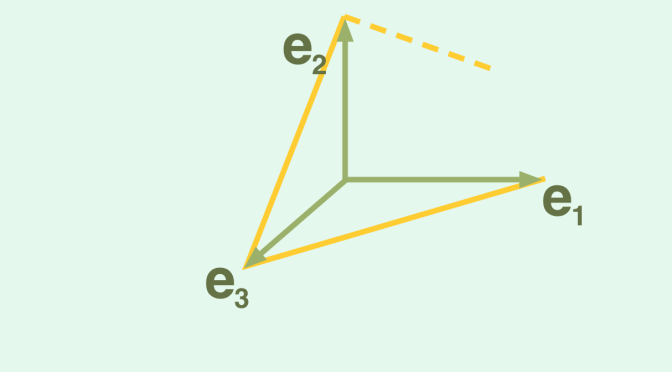# A solution of a differential equation not exploding in finite time

In this post, I mention that Peano existence theorem is valid for finite dimensional vector spaces, but not for Banach spaces of infinite dimension. I highlight here a second property of ordinary differential equations which is valid for finite dimensional vector spaces but not for infinite dimensional Banach spaces.

We consider a Banach space $$E$$, a real interval $$I$$ with $$t_0$$ as right endpoint and a function $$\textbf{f}$$ defined on $$I \times E$$ with codomain $$E$$ having following properties:

• $$\textbf{f}$$ is continuous.
• $$\textbf{f}$$ is locally Lipschitz continuous which means that for every point $$(t,\textbf{x}) \in I \times E$$ we can find a neighborhood $$V$$ of $$t$$, a neighborhood $$W$$ of $$\textbf{x}$$ and a number $$k>0$$ such that $$\Vert \textbf{f}(s,\textbf{x}_1)-\textbf{f}(s,\textbf{x}_2) \Vert \le k \Vert \textbf{x}_1 – \textbf{x}_2 \Vert$$ for all $$s \in V$$ and all $$\textbf{x}_1,\textbf{x}_2 \in W$$.

With above conditions met and according to the Picard–Lindelöf theorem, the differential equation $$\textbf{x}^\prime = \textbf{f}(t,\textbf{x})$$ is having a unique maximal solution $$\textbf{u}$$ to the initial value problem $$\textbf{x}(t)=\textbf{x}_0$$ defined on an interval $$J \subseteq I$$.

If $$J \neq I$$ and $$E$$ of finite dimension, one can prove that $$\lim\limits_{t \rightarrow a^+} \textbf{u}(t) = + \infty$$ where $$a$$ is $$J$$ lower endpoint. This might not be the case when $$E$$ if of infinite dimension. Following counterexample is from the French mathematician Jean Dieudonné.

We pick up for $$E$$ the Banach space of real sequences converging to $$0$$ for the norm $$\Vert \textbf{x} \Vert = \sup_n \vert x_n \vert$$ and define as $$\textbf{e}_n$$ the sequence whose terms are all vanishing except the one of index $$n$$ whose value is equal to $$1$$. For all $$n \in \mathbb{N}$$, we define the map from $$E$$ to $$E$$:
$\textbf{f}_n(\textbf{x})=[2(\textbf{x}_n+\textbf{x}_{n+1})-1]^+(\textbf{e}_{n+1}-\textbf{e}_{n})$ with $$\textbf{x}=(x_m) \in E$$.
$$\textbf{f}_n$$ is lipschitz continuous, vanishes on the closed ball $$\Vert \textbf{x} \Vert \le \frac{1}{4}$$ and $$\textbf{f}_n(x)=\textbf{e}_{n+1}-\textbf{e}_n$$ for $$\textbf{x}=\lambda \textbf{e}_n + (1-\lambda) \textbf{e}_{n+1}$$ where $$\lambda$$ is any real. For all integers $$n > 0$$, we pick up a continuous positive map $$\varphi_n$$ defined on the interval $$[\frac{1}{n+1},\frac{1}{n}]$$, vanishing at the endpoints of this interval and such that $$\displaystyle \int_{\frac{1}{n+1}}^{\frac{1}{n}} \varphi_n(t) dt=1$$.

Let $$I=(-\infty,1]$$ and define the map:
$\begin{array}{l|rcl} \textbf{f} : & I \times E & \longrightarrow & E\\ & (t,\textbf{x}) & \longmapsto & \textbf{f}(t,\textbf{x})=\textbf{0} \text{ for } t \le 0\\ & (t,\textbf{x}) & \longmapsto & \textbf{f}(t,\textbf{x})=\varphi_n(t)\textbf{f}_n(x) \text{ for } t \in [\frac{1}{n+1},\frac{1}{n}]\end{array}$
It is clear that $$\textbf{f}$$ is continuous and locally Lipschitz continuous at all points $$(t_0,\textbf{x}_0) \in I \times E$$ for $$t_0 \neq 0$$. We prove that the assertion is also true at all points $$(0,\textbf{a})$$ with $$\textbf{a}=(a_n)$$. Because $$\textbf{a}$$ converges to zero, one can find $$m \in \mathbb{N}$$ such that $$\vert a_n \vert \le \frac{1}{8}$$ for $$n \ge m$$. Hence for $$\textbf{x} \in E$$ and $$\Vert \textbf{x} – \textbf{a} \Vert \le \frac{1}{8}$$ we get $$\vert x_n \vert \le \frac{1}{4}$$ for $$n \ge m$$ and therefore $$\textbf{f}_n(\textbf{x})=\textbf{0}$$ for all $$n \ge m$$. Finally, $$\textbf{f}(t,\textbf{x})$$ vanishes for $$t \in [0,\frac{1}{m}]$$ and $$\Vert \textbf{x} – \textbf{a} \Vert \le \frac{1}{8}$$ leading to the expected conclusion.

We now define a sequence (for $$n \ge 1$$) of maps $$\textbf{v}_n: I\ \mapsto E$$:
$\textbf{v}_1(t) = \begin{cases} 0 & \text{ for } t < \frac{1}{2}\\ \textbf{e}_1+(\textbf{e}_2-\textbf{e}_1) \int_t^1 \varphi_1(s) ds & \text{ for } \frac{1}{2} \le t \le 1 \end{cases}$ and for $$n \ge 2$$: $\textbf{v}_n(t) = \begin{cases} 0 & \text{ for } t < \frac{1}{n+1} \text{ or } t > \frac{1}{n}\\ (\textbf{e}_{n+1}-\textbf{e}_n) \int_t^{\frac{1}{n}} \varphi_n(s) ds & \text{ for } \frac{1}{n+1} \le t \le \frac{1}{n} \end{cases}$ For $$0 < t \le 1$$, the series $$\textbf{u}(t) = \displaystyle \sum_{n=1}^\infty \textbf{v}_n(t)$$ is eventually vanishing and therefore well defined. For $$\frac{1}{n+1} \le t \le \frac{1}{n}$$ we have $$\textbf{u}(t)=\textbf{e}_n+(\textbf{e}_{n+1}-\textbf{e}_n) \int_t^{\frac{1}{n}} \varphi_n(s) ds$$ which implies that $$\Vert \textbf{u}(t) \Vert \le 1$$ for $$0 < t \le 1$$, and is differentiable in this interval. Considering the definition of $$\textbf{f}$$ we get $$\textbf{u}^\prime(t)=-\textbf{f}(t,\textbf{u}(t))$$. We also notice that $$\textbf{u}(1/n)=\textbf{e}_n$$ for all positive integers. Hence, $$\textbf{u}(t)$$ has no limit when $$t$$ tends to $$0$$. Consequently $$\textbf{u}$$ is a solution of the initial value problem $$\textbf{x}^\prime(t)=-\textbf{f}(t,\textbf{x}(t))$$ and $$\textbf{x}(1)=\textbf{e}_1$$. $$J=(0,1]$$ is the maximal interval of the solution $$\textbf{u}$$. We have proven that $$J \neq I$$ while $$\textbf{u}(t)$$ remains bounded on $$J$$ as desired.

## 2 thoughts on “A solution of a differential equation not exploding in finite time”

1.Tadashi says:

Dear Jean-Pierre Merx,

Thanks for another enlightening example! 🙂
I think there is a typo in the definition of $v_n$ for $n \ge 2$: instead of $\varphi_1$ shouldn’t be $\varphi_n$?

Thanks and best regards!

1.Jean-Pierre Merx says: